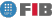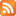# Stochastic Models for the Operative Investigation (MEIO)

Credits Dept. Type Requirements
7.5 (6.0 ECTS) EIO
• Elective for DIE
• Elective for DCSYS
EST - Prerequisite for DIE , DCSFW

## Instructors

 Person in charge: (-) Others: (-)

## General goals

By modelling them, students are introduced to the operations research techniques used in analysing systems for quantitative decision making in the presence of uncertainty.

## Specific goals

### Knowledges

1. The main non-deterministic mathematical models for analyzing systems in the presence of uncertainty: queuing networks, reliability, availability, simulating queues and reliability.
2. The methodology for the construction of these models.
3. The algorithms for treating them and their computing implementation.
4. Procedures for analyzing and interpreting solutions.
5. The application of these models to the evaluation of computing systems.

### Abilities

1. System modelling where randomness is present.
2. Ability to apply models of these systems to decision- making in applications that involve queues, reliability, etc.
3. Ability to apply methods for analyzing systems in the presence of uncertainty:
4. Ability to analyze the solutions provided by the models, and to draw conclusions.

### Competences

1. Ability to solve problems through the application of scientific and engineering methods.
2. Understanding scientific method.
3. Ability to create and use models of reality.
4. Ability to design and carry out experiments and analyse the results.

## Contents

Estimated time (hours):

 T P L Alt Ext. L Stu A. time Theory Problems Laboratory Other activities External Laboratory Study Additional time

1. Introduction
T      P      L      Alt    Ext. L Stu    A. time Total
2,0 0 0 0 0 1,0 0 3,0
Basic elements in the methodology for constructing models of systems modelling systems under conditions of uncertainty.

2. Basic models of Queuing Theory
T      P      L      Alt    Ext. L Stu    A. time Total
15,0 8,0 0 0 0 15,0 0 38,0
a. General structure of queuing models.

b. Queuing models based on birth-death processes.

c. Generalised queuing models with non-exponential distributions.

d. Exponential queuing models in series.

e. Introduction to the basic elements and concepts in the analysis of Markovian processes.

f. Queue networks: open and closed networks. Markovian queues. Introduction to general service distributions and multiple job types.

3. Applications of Queuing Theory
T      P      L      Alt    Ext. L Stu    A. time Total
4,0 4,0 0 0 0 8,0 0 16,0

a. Examples.

b. Decision-making processes in analyzing systems based on queuing models

c. Formulation of cost functions based on queuing models

d. System analysis models. Applications of open networks (with and without feedback) and closed networks to the modelling of a central computer server. Extensions to the generalisation of service time and multiplicity of job types.

4. Introduction to System Reliability and Availability models.
T      P      L      Alt    Ext. L Stu    A. time Total
6,0 4,0 0 0 0 10,0 0 20,0

a. Statistical models concerning reliability. Graphic methods.

b. Standard fault prediction and modelling methods: basic series, active redundancy.

c. Availability models for reparable systems. Availability and maintenance cost: Modular design. Analysis, using block diagrams and Markovian processes. Simulation limitations and treatment.

5. Introduction to Queuing Simulation Models, Reliability and System Availability.
T      P      L      Alt    Ext. L Stu    A. time Total
20,0 2,0 14,0 0 10,0 20,0 0 66,0

a. Introduction to system simulations: simulation event scheduling.

b. Basic simulation models for queues, reliability, etc.

c. Introduction to Monte Carlo methods: generation of random numbers and samples of probability distributions.

d. Introduction to simulation experiments and analysis of simulation results.

 Total per kind T P L Alt Ext. L Stu A. time Total 47,0 18,0 14,0 0 10,0 54,0 0 143,0 Avaluation additional hours 7,0 Total work hours for student 150,0

## Docent Methodolgy

The course will take a practical approach with regard to the application of models, especially in those cases where models provide the most efficient way of representing and analyzing computing systems (e.g. queues and queue networks).

The teaching approach adopted in the course combines theory classes, problems, and lab sessions. This is complemented by a small project in which students must build a model for analyzing and evaluating system behaviour.

## Evaluation Methodgy

Course assessment is based on the following:

1. A test (C1) will be held halfway through the term in class time.

2. A test (C2) will be held before the end of term and in class time.

3. Simulation course (C3). This will be carried out during the lab sessions and involve individual work at an external lab.

4. The final exam will comprise two parts directly bearing on the materials examined in tests C1 an C2, (following on from C4-1 and C4-2).

The Continuous Assessment grade and Final Exam grade will be determined as follows:

Continuous Assessment = 0.5*C1+0.5*C2

Final Exam = 0.5*Max(C1,C4-1)+0.5*Max(C2,C4-2)

Award of the Final Grade (NF) will require submissions of the course practical work (C3) and be calculated as follows:

NF = 0.8*Max(NAC,NEF)+0.2*C3

The maximum Final Grade is 4.

Students who pass both the two interim tests may sit the final exam if they so wish.

## Basic Bibliography

• Sanjay K. Bose. An Introduction to queueing systems, Kluwer Academic/Plenum Publishers, 2002.
• Frederick S. Hillier, Gerald J. Lieberman Introduction to operations research, McGraw-Hill, 1995.
• Hisashi Kobayashi Modeling and analysis : an introduction to system performance evaluation methodology, Addison-Wesley, 1978.
• Stephen S. Lavenberg, [editors] Computer performance modeling handbook, Academic Press, 1983.
• Wayne L. Winston Operations research : applications and algorithms, Brooks/Cole - Thomson Learning, 2004.

## Complementary Bibliography

• Averill M. Law. Simulation modeling and analysis, McGraw-Hill, 2007.
• P. Bratley, B.l. Fox and L.E. Schrage A Guide to Simulation, Springer-Verlag, 1987.
• Arnold O. Allen Probability, statistics and queueing theory : with computer science applications, Academic Press, 1990.
• Kishor Shridharbhai Trivedi Probability and statistics with reliability, queuing and computer science applications, John Wiley & Sons, 2002.
• Patrick D.T. O'Connor; with David Newton, Richard Bromley Practical reliability engineering, Wiley, 1995.
• Raj Jain The Art of computer systems performance analysis : techniques for experimental design, measurement, simulation, and modeling, John Wiley & Sons, 1991.

1. http://www-eio.upc.es/fme/teaching/mioas/index.html

2. http://www.ifors.org/

3. http://www.euro-online.org/

4. http://www.informs.org/

## Previous capacities

Algebra, Analysis, Statistics© Barcelona school of informatics - Contact -RSS
This website uses cookies to offer you the best experience and service. If you continue browsing, it is understood that you accept our cookies policy.
Classic version Mobile version